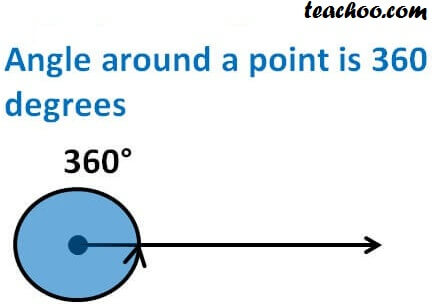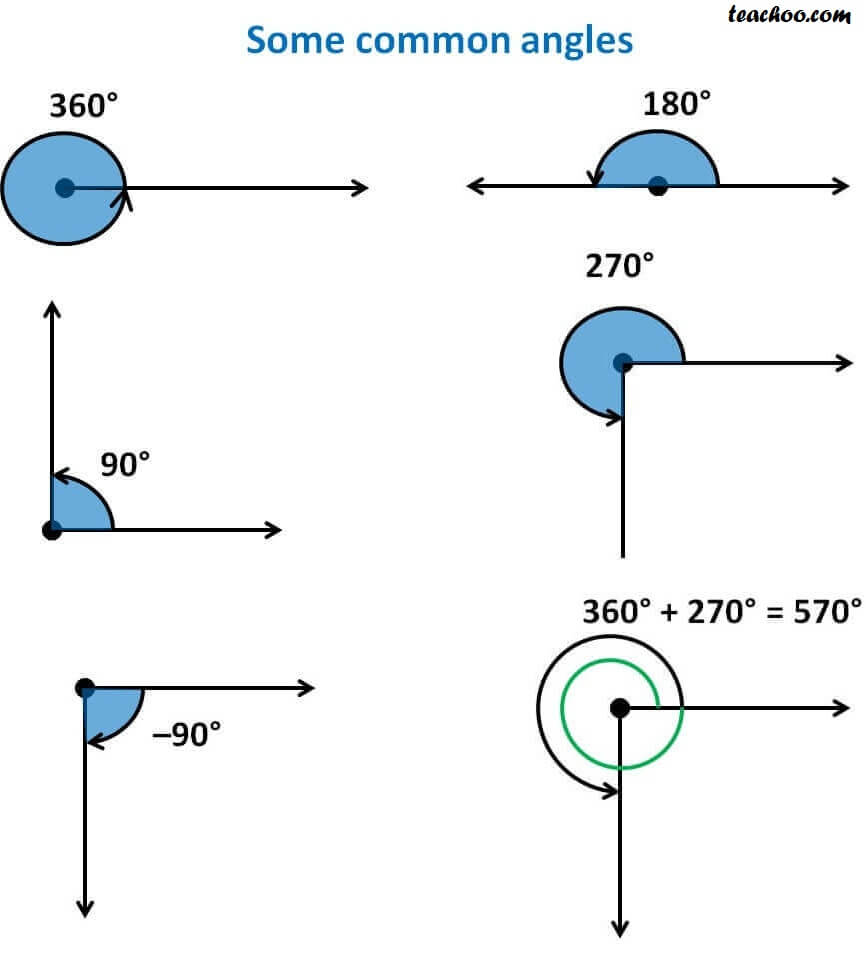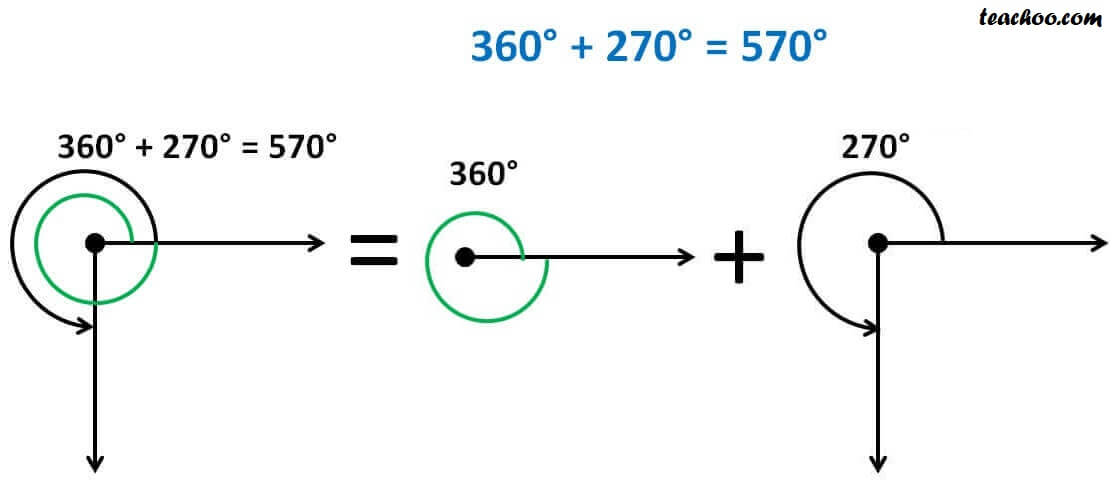Chapter 3 Class 11 Trigonometric Functions
Concept wise

Degree is used for measuring angles

Angle around a point is 360 degrees i.e. 360°

Symbol for degree is °Now,

60 minutes is equal to 1 degree

60 minutes = 1°

60’ = 1°

1’ = 1/60 °

And

60 seconds is equal 1 minute

60 seconds = 1’

60’’  = 1’

1’’ = 1/60 ‘

## Some common angles areNote

Angles are positive when it is measured anti-clockwise

Learn in your speed, with individual attention - Teachoo Maths 1-on-1 Class

### Transcript

Degree is used for measuring angles Angle around a point is 360 degrees i.e. 360 Symbol for degree is Now, 60 minutes is equal to 1 degree 60 minutes = 1 60 = 1 1 = 1/60 And 60 seconds is equal 1 minute 60 seconds = 1 60 = 1 1 = 1/60 Some common angles are Note Angles are positive when it is measured anti-clockwise